# My OLLs## Kevin Guillaumond

December 25, 2020

This is part two of my series of posts detailing my cubing algorithms: the OLLs.

There are 57 OLL cases but I sometimes have variations to influence the PLL, either to put the corners in the correct position, or at least to avoid a diagonal PLL (ENVY). That makes 71 sequences in total.

“COLL case” means that after the sequence is performed, the corners will be in the correct position.

“Diag case” means that after the sequence is performed, two opposite corners will be swapped, leaving an E, N, V or Y PLL.

# Corners only

Case Image Algorithm Comment
26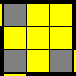(R U R' U) (R U2 R') The Sune. You can also do an Antisune with your left hand
27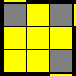(R U2) (R' U' R U' R') The Antisune. You can also do a Sune with your left hand
21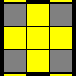R U2' R' U' R U R' U' R U' R' Two Antisunes in a row, with annulation. COLL when there are two vertical bars
23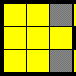(R U2' R' U' R U' R') (L' U2 L U L' U L) Right antisune + Left antisune
24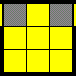Lw' U' R D' R' U R D x' COLL case when UBL/UBR are adjacent and LUF/UBR the same (or RUF/UBL different)
25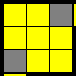Lw' U R D' R' U' R D x' Very similar to the previous one. You can probably use the symmetric to avoid ENVY but I don’t use it
22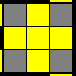R U2' R2' U' R2 U' R2' U2' R COLL case when URF/URB are the same and ULF/ULB different

## Alternatives

Case Image Algorithm Comment
23(R U2' R' U' R U' R') U2 (R' U2' R U R' U R) Same as the defaut one but all RU
23(R U2' R' U' R U' R') U' (R U' L' U R' U' L) Antisune + Niklas. COLL case when URB/URF are adjacent colors and FUL is adjacent to ULB
23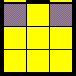R2' D' R U2 R' D R U2 R COLL case when ULB/URB are the same color and FUL is the same color as ULB
23R' F (R U' R' U') (R U R' F') (R U R' U') (R' F R F') R Y perm with an R' setup. COLL case when ULB/URB are the same color and RUF is adjacent
21F (R U R' U')3 F' COLL when there are two horizontal bars, which is the diag case with the normal sequence
24Rw U L' D L U' L' D' x' Symetric of the other sequence, so it is the COLL when UBL/UBR are adjacent and RUF/UBL the same (the other sequence would result in ENVY)
22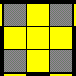(Rw U R' U) (R' F R F') (R U' R' U) (R U2 Rw') This is for the diag case (if the bar is on the left instead of the right). This is the COLL.
22R' U2' R2 U R2' U R2 U2' R ZBLL time! This is the F/B mirror of the normal sequence, used in the COLL case when FU is the opposite color of URF/URB. This avoid a Z perm (so forces U, U', H or Skip)

# Bars, part 1: Ts, Cs, and big lignthing bolts

Case Image Algorithm Comment
33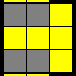(R U R' U') (R' F R F') Beginning of the T perm. COLL case when ULF/ULB different and FUR/ULB same. If different, do U2 + symmetric sequence with your left hand to avoid ENVY and get the COLL
45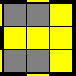F (R U R' U') F'
46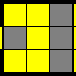(R' U') (R' F R F') (U R)
34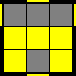R U R2' U' R' F R U R U' F'
40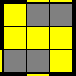(R' F) (R U R' U') F' U R
39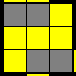(L F') (L' U' L U) F U' L' L/R mirror of the previous one

# Bars, part 2: Just the bar

Case Image Algorithm Comment
51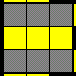F (U R U' R')2 F'
52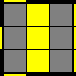(R' U' R U' R' Dw) (R' U R B) The F/B mirror also works
56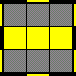F (R U R' U') R F' Rw U R' U' Rw'
55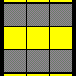Rw U2 R' U' R Rw' (R U R' U') Rw U' Rw' Sexy move inside a Fat Antisune, similar to #54

# Bars, part 3: Big Ls

Case Image Algorithm Comment
16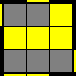(Rw U Rw') (R U R' U') (Rw U' Rw') Sexy move with a setup
15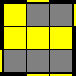(Lw' U' Lw) (L' U' L U) (Lw' U Lw) L/R mirror of the previous one
13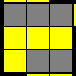Rw U' Rw' U' Rw U Rw' y' R' U R
14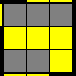Lw' U Lw U Lw' U' Lw y L U' L' L/R mirror of the previous one

# Adjacent edges, part 1: Small Ls

Case Image Algorithm Comment
48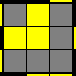F (R U R' U')2 F'
47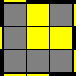F' (L' U' L U) F L/R mirror of the previous one
54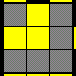Rw U2 R' U' (R U R' U') R U' Rw' Sexy move inside a Fat Antisune, similar to #55
53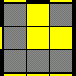Lw' U2' L U (L' U' L U) L' U Lw L/R mirror of the previous one
49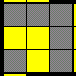R B' R2' F R2 B R2' F' R
50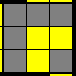Rw' U Rw2 U' Rw2' U' Rw2 U Rw' Actually the L/R mirror of the previous one, but written in a more right-handed friendly way

# Adjacent edges, part 2: Fishes and squares

Case Image Algorithm Comment
37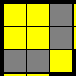F (R U' R' U') (R U R' F') First half of the Y perm
35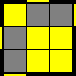R U2 R2 F R F' R U2 R'
10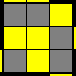(R U R' U) (R' F R F') (R U2 R')
9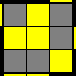(R U R' U' R' F) (R2 U R' U' F')
6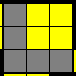Rw U2 R' U' R U' Rw' Fat Antisune
5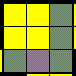Lw' U2' L U L' U Lw Fat Antisune on the left hand

# Adjacent edges, part 3: Ps and Ws

Case Image Algorithm Comment
44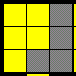F (U R U' R') F'
43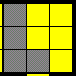F' (U' L' U L) F
31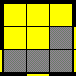(F R' F' R) (U R U R') (U' R U' R')
32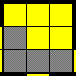(F' L F L') (U' L' U' L) (U L' U L) L/R mirror of the previous one
38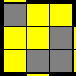(R U R' U) (R U' R' U') (R' F R F') Inverse of #31
36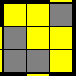(L' U' L U') (L' U L U) (L F' L' F) L/R mirror of #38 and inverse of #32

## Alternatives

Case Image Algorithm Comment
44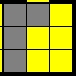Fw (R U R' U') Fw' Avoids an initial U2
43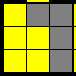Fw' (L' U' L U) Fw Avoids an initial U2

# Adjacent edges, part 3: Awkward shapes

Case Image Algorithm Comment
41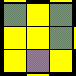(R U R' U R U2' R') F (R U R' U') F' Two known sequences in a row
42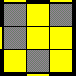(L' U' L U' L' U2 L) F' (L' U' L U) F L/R mirror of the previous one
29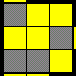(R U R' U') (R U' R') (F' U' F) (R U R')
30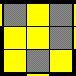F U (R U2 R' U')2 F'

# Dots

Case Image Algorithm Comment
2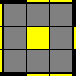F (R U R' U') S (R U R' U') Fw'
1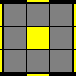(R U2) (R2' F R F') U2' (R' F R F')
19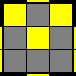R Rw' U (R U R' U') Rw R2' F R F' Similar to #20
18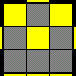Rw U R' U R U2 Rw2' U' R U' R' U2 Rw
17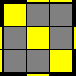(R U R' U) (R' F R F') U2 (R' F R F')
3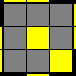Fw (R U R' U') Fw' U' F (R U R' U') F' Yellow corner on right side + yellow bar on R
4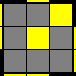Fw (R U R' U') Fw' U F (R U R' U') F' Same as before, only the middle move changes direction

# Edges only

Case Image Algorithm Comment
28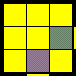(Rw U R' U') M' (U R U' R')
57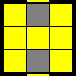(R U R' U') M (U R U' Rw') Inverse of the previous one
20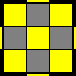R Rw' U (R U R' U') Rw2 R2' U R U' Rw' The least probable case (1/216). Similar to #19

## Alternatives (all for the pure orientation)

Case Image Algorithm Comment
28(Rw U R' U') Rw' U2 R U R U' R2' U2' R
57M' U M' U M' U2 M U M U M U2
20(M' U)4 (M U)4